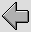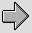Virtually every electronic circuit requires some form of power supply. This normally means that it requires one or more Power Rails – lines held at specific steady d.c. voltages – from which the required current and hence power may be drawn. There is also usually a need for an Earth connection, both as a reference level and for safety purposes. In some cases the power can come from a battery or some other kind of chemical cell. However most systems require a Power Supply Unit (PSU) that can take a.c. power from the mains and convert this into the required d.c. levels. This lecture will examine the basic types of mains-to-d.c. analog PSUs that are used and consider their main properties.

The basic PSU
Figure 5·1 illustrates the traditional basic form of a simple mains PSU. It consist of a Transformer, a diode, and a smoothing or Reservoir capacitor.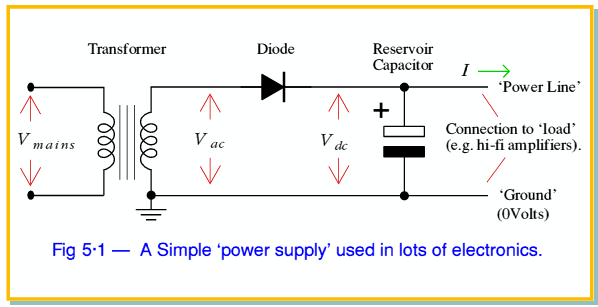We will assume that we don’t need to consider the details of how diodes and capacitors work at this point. Instead we can just make a few relevant comments. The most important property of a diode in the context of a PSU is that it will conduct current when a Forward Bias is applied, but refuses to conduct when a Reverse Bias is applied. Hence when the output from the transformer means that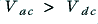the diode will pass current and the capacitor will be charged up by the applied voltage from the transformer. However when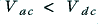the diode will refuse to conduct. Hence none of the charge in the capacitor will be removed again via the diode. Instead it will be available to the output load and the capacitor can supply current to the amplifiers, etc, which use the PSU output. in practice, there will always be a modest voltage drop across the diode when it conducts, so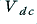will never be as large as the peak positive value of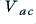.

Note that the capacitor shown in figure 6·1 is an Electrolytic type. In theory this isn’t essential. However we often find that we want very large capacitance values (perhaps tens or hundreds of thousands of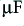) and these would become physically very large and expensive unless we choose electrolytics. Electrolytic capacitors have a number of properties which make them undesirable in high-performance circuits, and can explode if used ‘the wrong way around’ or at too high a voltage. However they offer large amounts of capacitance in a given volume for a given cost so are widely used in PSUs.

Figure 5·2 illustrates how the voltages and current in the circuit behave in a typical situation.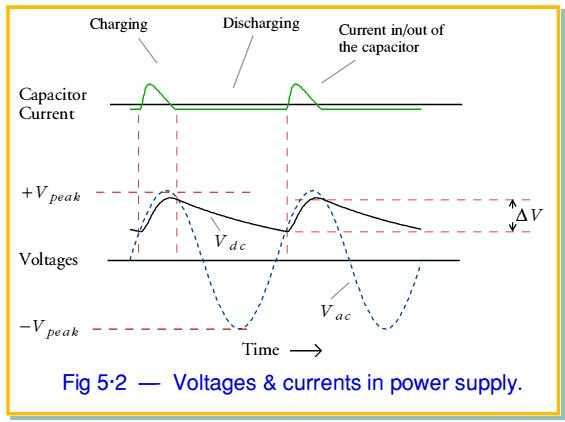The input transformer ‘steps down’ the mains input to provide a 50Hz sinewave voltage [I am assuming we are in the United Kingdom, hence have 50Hz mains power],, which swings up & down over the range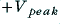to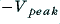as shown in figure 5·2. The value of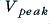depends on the turns ratio of the transformer. The voltage on the capacitor,, at any moment depends on how much charge it holds.

Whenthe diode is forward biassed. Current then flows through the diode, charging the capacitor. Asrises toduring each cycle it pumps a pulse of charge into the capacitor & lifts. Using a silicon diode we can expect the peak value ofto approximately be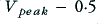Volts since the diode always ‘drops’ around half a volt when it’s conducting. Whenswings belowthe diode stops conducting & blocks any attempt by charge in the capacitor to get out again via the diode. Since the mains frequency is 50Hz these recharging pulses come 50 times a second.

In the gaps between recharging pulses the dc current drawn out of the supply will causeto fall. How much it falls during these periods depends upon the amount of current drawn & the size of the capacitor. The time between pulses will be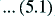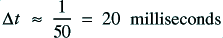During this time a current,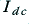, would remove a charge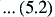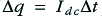from the capacitor, reducing the d.c. voltage by an amount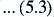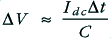before it can be lifted again by the next recharging pulse.

As a result of this repetitive charging/discharging process during each mains cycle the output voltage ripples up & down by an amount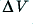. Hence the output isn't a perfectly smooth d.c. voltage. However, from equation 5.3 we can see that it's possible to reduce the amount of ripple by choosing a larger value capacitor for the reservoir, C. This is why PSUs tend to use large capacitances – it minimises the amount of ripple. Ideally, a PSU will provide a fixed d.c. level, but in reality it will have ripple, and may alter when the current demanded by the load changes. In general a ‘better’ supply will provide a more stable, constant output d.c. level.